Hostname: page-component-797576ffbb-6mkhv Total loading time: 0 Render date: 2023-12-03T08:49:55.918Z Has data issue: false Feature Flags: { "corePageComponentGetUserInfoFromSharedSession": true, "coreDisableEcommerce": false, "useRatesEcommerce": true } hasContentIssue false

# ON THE EXPONENTIAL DIOPHANTINE EQUATION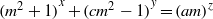$\mathop{({m}^{2} + 1)}\nolimits ^{x} + \mathop{(c{m}^{2} - 1)}\nolimits ^{y} = \mathop{(am)}\nolimits ^{z}$

Published online by Cambridge University Press:  26 March 2014

## Abstract

Core share and HTML view are not possible as this article does not have html content. However, as you have access to this content, a full PDF is available via the ‘Save PDF’ action button.

Let$m$,$a$,$c$ be positive integers with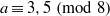$a\equiv 3, 5~({\rm mod} \hspace{0.334em} 8)$. We show that when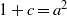$1+ c= {a}^{2}$, the exponential Diophantine equation$\mathop{({m}^{2} + 1)}\nolimits ^{x} + \mathop{(c{m}^{2} - 1)}\nolimits ^{y} = \mathop{(am)}\nolimits ^{z}$ has only the positive integer solution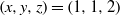$(x, y, z)= (1, 1, 2)$ under the condition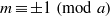$m\equiv \pm 1~({\rm mod} \hspace{0.334em} a)$, except for the case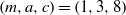$(m, a, c)= (1, 3, 8)$, where there are only two solutions: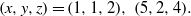$(x, y, z)= (1, 1, 2), ~(5, 2, 4).$ In particular, when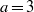$a= 3$, the equation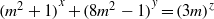$\mathop{({m}^{2} + 1)}\nolimits ^{x} + \mathop{(8{m}^{2} - 1)}\nolimits ^{y} = \mathop{(3m)}\nolimits ^{z}$ has only the positive integer solution$(x, y, z)= (1, 1, 2)$, except if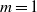$m= 1$. The proof is based on elementary methods and Baker’s method.

Type
Research Article
Information

## References

Bennett, M. A., ‘On some exponential equations of S. S. Pillai’, Canad. J. Math. 53 (2001), 897922.Google Scholar
Bosma, W. and Cannon, J., ‘Handbook of Magma functions’, School of Mathematics and Statistics, University of Sydney, available at http://magma.maths.usyd.edu.au/magma/.Google Scholar
Bugeaud, Y., ‘Linear forms in$p$-adic logarithms and the Diophantine equation$({x}^{n} - 1)/ (x- 1)= {y}^{q}$’, Math. Proc. Cambridge Philos. Soc. 127 (1999), 373381.Google Scholar
Cao, Z. and Dong, X., ‘An application of a lower bound for linear forms in two logarithms to the Terai–Jeśmanowicz conjecture’, Acta Arith. 110 (2003), 153164.Google Scholar
He, B. and Togbé, A., ‘The Diophantine equation${n}^{x} + \mathop{(n+ 1)}\nolimits ^{y} = \mathop{(n+ 2)}\nolimits ^{z}$ revisited’, Glasg. Math. J. 51 (2009), 659667.Google Scholar
He, B. and Togbé, A., ‘On the positive integer solutions of the exponential Diophantine equation${a}^{x} + \mathop{(3{a}^{2} - 1)}\nolimits ^{y} = \mathop{(4{a}^{2} - 1)}\nolimits ^{z}$’, Adv. Math. (China) 40 (2011), 227234.Google Scholar
He, B. and Yang, S., ‘The solutions of a class of exponential Diophantine equation’, Adv. Math. (China) 41 (2012), 565573.Google Scholar
Jeśmanowicz, L., ‘Some remarks on Pythagorean numbers’, Wiad. Mat. 1 (1955/1956), 196202 (in Polish).Google Scholar
Khinchin, A. Y., Continued Fractions, 3rd edn (P. Noordhoff, Groningen, 1963).Google Scholar
Laurent, M., ‘Linear forms in two logarithms and interpolation determinants II’, Acta Arith. 133 (2008), 325348.Google Scholar
Le, M. H., ‘On the exponential Diophantine equation$\mathop{({m}^{3} - 3m)}\nolimits ^{x} + \mathop{(3{m}^{2} - 1)}\nolimits ^{y} = \mathop{({m}^{2} + 1)}\nolimits ^{z}$’, Publ. Math. Debrecen 58 (2001), 461466.Google Scholar
Miyazaki, T., ‘Exceptional cases of Terai’s conjecture on Diophantine equations’, Arch. Math. (Basel) 95 (2010), 519527.Google Scholar
Miyazaki, T., ‘Terai’s conjecture on exponential Diophantine equations’, Int. J. Number Theory 7 (2011), 981999.Google Scholar
Miyazaki, T. and Togbé, A., ‘The Diophantine equation$\mathop{(2am- 1)}\nolimits ^{x} + \mathop{(2m)}\nolimits ^{y} = \mathop{(2am+ 1)}\nolimits ^{z}$’, Int. J. Number Theory 8 (2012), 20352044.Google Scholar
Nagell, T., ‘Contributions to the theory of a category of Diophantine equations of the second degree with two unknowns’, Nova Acta Reg. Soc. Upsal. IV Ser. 16 (2) (1955), 138.Google Scholar
Nagell, T., ‘Sur une classe d’équations exponentielles’, Ark. Mat. 3 (1958), 569582.Google Scholar
Sierpiński, W., ‘On the equation${3}^{x} + {4}^{y} = {5}^{z}$’, Wiad. Mat. 1 (1955/1956), 194195 (in Polish).Google Scholar
Terai, N., ‘The Diophantine equation${a}^{x} + {b}^{y} = {c}^{z}$’, Proc. Japan Acad. Ser. A Math. Sci. 70 (1994), 2226.Google Scholar
Terai, N., ‘Applications of a lower bound for linear forms in two logarithms to exponential Diophantine equations’, Acta Arith. 90 (1999), 1735.Google Scholar
Terai, N., ‘On the exponential Diophantine equation$\mathop{(4{m}^{2} + 1)}\nolimits ^{x} + \mathop{(5{m}^{2} - 1)}\nolimits ^{y} = \mathop{(3m)}\nolimits ^{z}$’, Int. J. Algebra 6 (2012), 11351146.Google Scholar